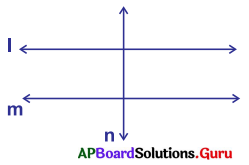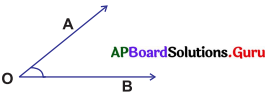# AP Board 7th Class Maths Solutions Chapter 4 Lines and Angles Review Exercise

SCERT AP 7th Class Maths Solutions Pdf Chapter 4 Lines and Angles Review Exercise Questions and Answers.

## AP State Syllabus 7th Class Maths Solutions 4th Lesson Lines and Angles Review Exercise

Question 1.
Observe the figure and name the points, line segments, rays, and lines from the figure.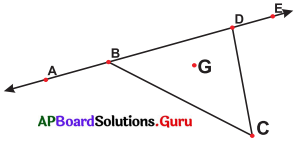Points: A, B, C, D, E, G
Line segments : $$\overline{\mathrm{AB}}, \overline{\mathrm{AD}}, \overline{\mathrm{AE}}, \overline{\mathrm{BD}}\overline{\mathrm{DE}}, \overline{\mathrm{BC}}, \overline{\mathrm{CD}}, \overline{\mathrm{BE}}$$
Rays : $$\overrightarrow{\mathrm{BA}}, \overrightarrow{\mathrm{DA}}, \overrightarrow{\mathrm{BE}}, \overrightarrow{\mathrm{AE}}, \overrightarrow{\mathrm{EA}}, \overrightarrow{\mathrm{DE}}$$
Lines : $$\overrightarrow{\mathrm{AE}}$$

Question 2.
Observe the figure and write intersect-ing lines and concurrent lines.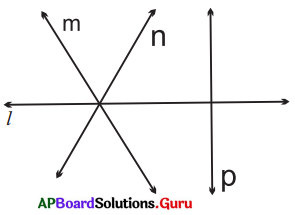Intersecting lines : l, p
Concurrent lines : l, m, nQuestion 3.
Draw a line segment PQ = 6.3 cm.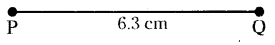Question 4.
Name any three possible angles in the adjacent figure.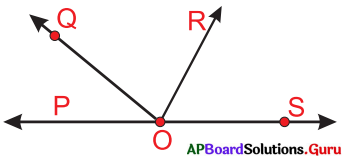Angles: ∠POQ, ∠POR, ∠POS, ∠OOS, ∠ROS, ∠QOR.

Question 5.
Write the type of angles you observed in the given clock.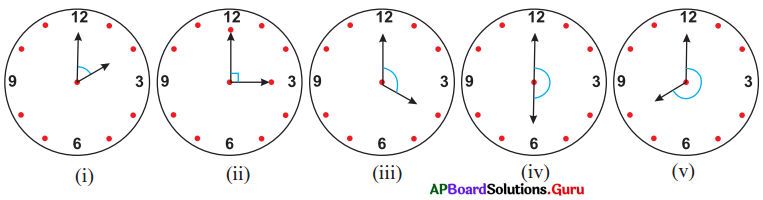Angles (i) Acute angle
(ii) Right angle
(iii) Obtuse angle
(iv) Straight angle
(v) Reflex angle.

Question 6.
One right angle is equal to __________ degrees.
90.

Question 7.
Write any two acute angles and any two obtuse angles.
Acute angles :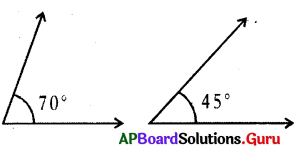Obtuse Angles: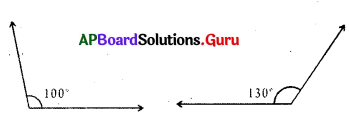Question 8.
Observe the parallel and perpendicular lines in the given figure. Write them using symbols ∥, ⊥